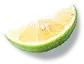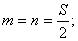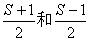# 小学奥数知识点趣味学习——整数的分拆（3）

2021-01-04 11 ℃23=15+（2+2+2+2），

23=15+（2+2+2+1+1），

23=15+（2+2+1+1+1+1），

23=20+（2+1），

23=20+（1+1+1），=2+7+11+17，共10种不同拆法，其中3×5×29=435最小。3=1+2，

4=1+3=2+2，

5=1+4=2+3，

6=1+5=2+4=3+3，

7=1+6=2+5=3+4，

8=1+7=2+6=3+5=4+4，

9=1+8=2+7=3+6=4+5，

11=1+10=2+9=3+8=4+7=5+6。

（1）当n为大于等于10的偶数时，n=2k=4+2（k-2）。由于n≥10，所以k≥5，k-2≥3，2（k-2）与4均为合数，且不相等。也就是说，大于等于10的偶数均能表示为两个不同的合数之和，应染红色。（1）当n为大于等于13的奇数时，

n=2k+1=9+2（k-4）。由于n≥13，所以k≥6，k-4≥2，2（k-4）与9均为合数，且不相等。也就是说，大于等于13的奇数均能表示为两个不同的合数之和，应染红色。